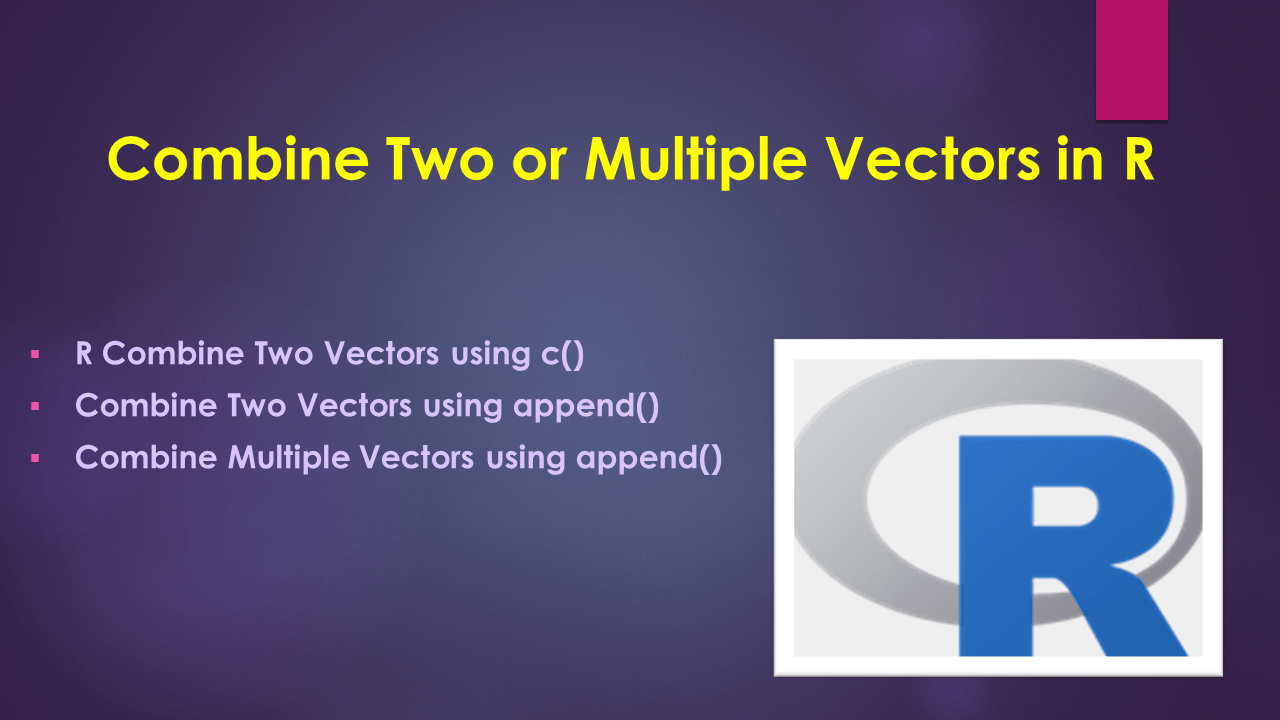# Combine Two or Multiple Vectors in R

To append two or more vectors into a single list or combine multiple vectors in R, you can use either the combine function c() or append() function. In this article, I will explain examples of using these two methods to combine multiple vectors in R.

Let’s create the three character vectors to use, I will define them as `vec1`, `vec2`, and `vec3`.

``````
# Create vectors to append
vec1 = c('sai','ram','deepika','sahithi')
vec2 = c('kumar','scott','Don','Lin')
vec3 = c('Ram','Denis')
``````

## 1. R Combine Two Vectors using c()

By using combine function c() you can append two vectors into a single vector in R. This function takes two vectors as an argument and returns the combined vector. The following example combines the elements from `vec1` and `vec2` objects into a single vector `vec4`.

Here c() is a combined function from the base package that can be used to combine lists, vectors, and other objects. These base functions are default available with the R installation and automatically loaded to the program.

``````
# combine two vectors
vec4 <- c(vec1,vec2)
print(vec4)
``````

Yields below output.

## 2. Combine Two Vectors using append()

Alternatively, you can also use append() to get two vectors into a single vector in R. This function also takes two vectors as an argument and returns the combined vector.

``````
# combine two vectors
vec4 <- append(vec1,vec2)
print(vec4)
``````

Yields the same output as above.

## 3. Combine Multiple Vectors using append()

Finally by using the append() let’s combine multiple vectors into a single vector in R. The below examples combine elements from `vec1`, `vec2` and `vec3` into a single vector `vec4`.

``````
# combine multiple vectors
vec4 <- c(vec1,vec2,vec3)
print(vec4)

vec4 <- append(vec1,vec2,vec3)
print(vec4)
``````

Yields below output.

## 4. Complete Example

Following is a complete example of appending two or multiple vectors.

``````
# create vectors
vec1 = c('sai','ram','deepika','sahithi')
vec2 = c('kumar','scott','Don','Lin')
vec3 = c('Ram','Denis')

# combine two vectors
vec4 <- c(vec1,vec2)
print(vec4)

# combine two vectors
vec4 <- append(vec1,vec2)
print(vec4)

# combine multiple vectors
vec4 <- c(vec1,vec2,vec3)
print(vec4)

vec4 <- append(vec1,vec2,vec3)
print(vec4)
``````

## 5. Conclusion

In this article, you have learned how to append two vectors into a single vector using the R c() combine function and append() function from R base package.

## Related Articles

### Naveen (NNK)

Naveen (NNK) is a Data Engineer with 20+ years of experience in transforming data into actionable insights. Over the years, He has honed his expertise in designing, implementing, and maintaining data pipelines with frameworks like Apache Spark, PySpark, Pandas, R, Hive and Machine Learning. Naveen journey in the field of data engineering has been a continuous learning, innovation, and a strong commitment to data integrity. In this blog, he shares his experiences with the data as he come across. Follow Naveen @ @ LinkedIn